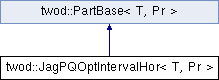# twod::JagPQOptIntervalHor< T, Pr > Class Template Reference

optimal PxQ jagged algorithm from "Ali Pinar and Cevdet Aykanat, Sparse Matrix Decomposition with Optimal Load Balancing, HiPC 1997" using the first dimension as the main dimension. More...

`#include <twod/jag_pq_opt_interval.hpp>`

Inheritance diagram for twod::JagPQOptIntervalHor< T, Pr >:## Detailed Description

### template<typename T, typename Pr> class twod::JagPQOptIntervalHor< T, Pr >

optimal PxQ jagged algorithm from "Ali Pinar and Cevdet Aykanat, Sparse Matrix Decomposition with Optimal Load Balancing, HiPC 1997" using the first dimension as the main dimension.

It uses oned::Nicol_plus_interval to partition the first dimension using an optimal partitioning algorithm in the second dimension. That way it gets an accurate value for the load of an interval.

The number of stripes in the main dimension can be changed using setP().

Parameters:
 T type of the data in the prefix sum array Pr type of the prefixsum array

List of all members.

## Public Member Functions

virtual ~JagPQOptIntervalHor ()
JagPQOptIntervalHor ()
void setP (int P)
selects the number of stripes in the main dimension
virtual T part (int procCount, const Pr &prefixSumArray, util::RectList< T, Pr > &parts)
optimal PxQ jagged algorithm from "Ali Pinar and Cevdet Aykanat, Sparse Matrix Decomposition with Optimal Load Balancing, HiPC 1997" using the first dimension as the main dimension.

## Static Private Member Functions

static T internal (int procX, int procY, const Pr &prefixSumArray, util::RectList< T, Pr > &parts)

int P

## Constructor & Destructor Documentation

template<typename T , typename Pr >
 twod::JagPQOptIntervalHor< T, Pr >::~JagPQOptIntervalHor ( ) ` [virtual]`
template<typename T , typename Pr >
 twod::JagPQOptIntervalHor< T, Pr >::JagPQOptIntervalHor ( )

## Member Function Documentation

template<typename T , typename Pr >
 T twod::JagPQOptIntervalHor< T, Pr >::internal ( int procX, int procY, const Pr & prefixSumArray, util::RectList< T, Pr > & parts ) ` [static, private]`
template<typename T , typename Pr >
 T twod::JagPQOptIntervalHor< T, Pr >::part ( int procCount, const Pr & prefixSumArray, util::RectList< T, Pr > & parts ) ` [virtual]`

optimal PxQ jagged algorithm from "Ali Pinar and Cevdet Aykanat, Sparse Matrix Decomposition with Optimal Load Balancing, HiPC 1997" using the first dimension as the main dimension.

It uses oned::Nicol_plus_interval to partition the first dimension using an optimal partitioning algorithm in the second dimension. That way it gets an accurate value for the load of an interval.

Parameters:
 [in] procCount number of processors in the partition. [in] prefixSumArray first column and first row consists of zero only. But the borders of rectangles in rect_list never touch this area (index 0 in row or column) [out] parts must be allocated before calling this function.
Returns:

Implements twod::PartBase< T, Pr >.

template<typename T , typename Pr >
 void twod::JagPQOptIntervalHor< T, Pr >::setP ( int P )

selects the number of stripes in the main dimension

Parameters:
 P number of stripes in the main dimension. If 0, the number of stripes will be computed at runtime by taking the square root of the number of processors.

## Member Data Documentation

template<typename T, typename Pr>
 int twod::JagPQOptIntervalHor< T, Pr >::P` [private]`

The documentation for this class was generated from the following files: